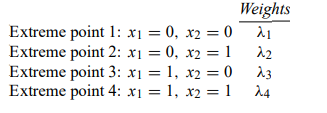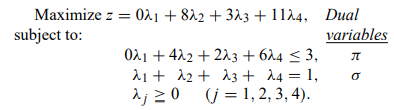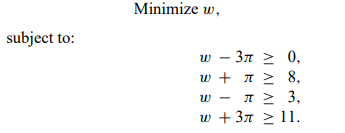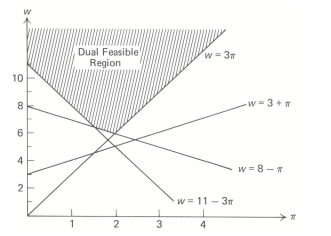# The decomposition method can be interpreted as a ‘‘cutting-plane’’ algorithm. To illustrate this viewpoint, consider the example

The decomposition method can be interpreted as a ‘‘cutting-plane’’ algorithm. To illustrate this viewpoint, consider the example:Applying decomposition with the constraints 0 ≤ x1 ≤ 1 and 0 ≤ x2 ≤ 1 as the subproblem, we have four extreme points to the subproblem:Evaluating the objective function 3×1 + 8×2 and resource usage 2×1 + 4×2 at these extreme-point solutions gives the following master problem:a) Let the variable w be defined in terms of the dual variables π and σ as w = σ + 3π. Show that the dual to the master problem in terms of w and π is:b) Figure depicts the feasible region for the dual problem. Identify the optimal solution to the dual problem in this figure. What is the value of z ∗ , the optimal objective value of the original problem?c) Suppose that we initiate the decomposition with a restricted master problem containing only the third extreme point x1 = 1 and x2 = 0. Illustrate the feasible region to the dual of this restricted master in terms of w and π, and identify its optimal solution w∗ and π ∗. Does this dual feasible region contain the feasible region to the full dual problem formulated in part (a)?

d) Show that the next step of the decomposition algorithm adds a new constraint to the dual of the restricted master problem. Indicate which constraint in Fig. is added next. Interpret the added constraint as ‘‘cutting away’’ the optimal solution w∗ and π ∗ found in part (c) from the feasible region. What are the optimal values of the dual variables after the new constraint has been added?

e) Note that the added constraint is found by determining which constraint is most violated at π = π ∗ ; that is, by moving vertically in Fig. at π = π ∗ , crossing all violated constraints until we reach the dual feasible region at w = ˆw. Note that the optimal objective value z ∗ to the original problem satisfies the inequalities:

w ∗ ≤ z ∗ ≤ ˆw

Relate this bound to the bounds discussed in this chapter.

f) Solve this problem to completion, using the decomposition algorithm. Interpret the solution in Fig., indicating at each step the cut and the bounds on z ∗

. g) How do extreme rays in the master problem alter the formulation of the dual problem? How would the cutting-plane interpretation discussed in this problem be modified when the subproblem is unbounded?